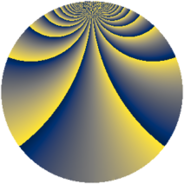# Properties

 Label 1045.2.vLevel $1045$ Weight $2$ Character orbit 1045.v Rep. character $\chi_{1045}(111,\cdot)$ Character field $\Q(\zeta_{9})$ Dimension $408$ Sturm bound $240$

# Related objects

## Defining parameters

 Level: $$N$$ $$=$$ $$1045 = 5 \cdot 11 \cdot 19$$ Weight: $$k$$ $$=$$ $$2$$ Character orbit: $$[\chi]$$ $$=$$ 1045.v (of order $$9$$ and degree $$6$$) Character conductor: $$\operatorname{cond}(\chi)$$ $$=$$ $$19$$ Character field: $$\Q(\zeta_{9})$$ Sturm bound: $$240$$

## Dimensions

The following table gives the dimensions of various subspaces of $$M_{2}(1045, [\chi])$$.

Total New Old
Modular forms 744 408 336
Cusp forms 696 408 288
Eisenstein series 48 0 48

## Trace form

 $$408 q + 12 q^{4} + 12 q^{6} + O(q^{10})$$ $$408 q + 12 q^{4} + 12 q^{6} + 12 q^{10} + 24 q^{12} + 12 q^{13} - 36 q^{16} - 24 q^{17} + 24 q^{19} + 36 q^{21} - 24 q^{23} - 48 q^{24} + 48 q^{27} + 72 q^{28} - 12 q^{31} - 120 q^{32} + 72 q^{34} - 72 q^{36} + 72 q^{38} - 96 q^{39} + 24 q^{40} - 48 q^{41} - 96 q^{42} + 84 q^{43} - 60 q^{46} - 12 q^{47} - 228 q^{49} + 48 q^{51} - 96 q^{52} - 96 q^{53} - 96 q^{54} + 36 q^{59} - 24 q^{60} - 24 q^{61} - 60 q^{62} - 156 q^{63} - 264 q^{64} + 60 q^{66} + 12 q^{67} - 12 q^{68} - 48 q^{69} - 36 q^{71} + 168 q^{72} + 156 q^{73} + 96 q^{74} - 24 q^{75} + 48 q^{76} + 132 q^{78} + 84 q^{79} - 96 q^{80} + 108 q^{82} - 96 q^{85} - 72 q^{86} + 120 q^{87} + 36 q^{88} + 120 q^{89} + 12 q^{90} - 168 q^{91} + 216 q^{92} - 48 q^{93} - 48 q^{94} + 456 q^{96} - 12 q^{97} + 192 q^{98} + O(q^{100})$$

## Decomposition of $$S_{2}^{\mathrm{new}}(1045, [\chi])$$ into newform subspaces

The newforms in this space have not yet been added to the LMFDB.

## Decomposition of $$S_{2}^{\mathrm{old}}(1045, [\chi])$$ into lower level spaces

$$S_{2}^{\mathrm{old}}(1045, [\chi]) \cong$$ $$S_{2}^{\mathrm{new}}(19, [\chi])$$$$^{\oplus 4}$$$$\oplus$$$$S_{2}^{\mathrm{new}}(95, [\chi])$$$$^{\oplus 2}$$$$\oplus$$$$S_{2}^{\mathrm{new}}(209, [\chi])$$$$^{\oplus 2}$$